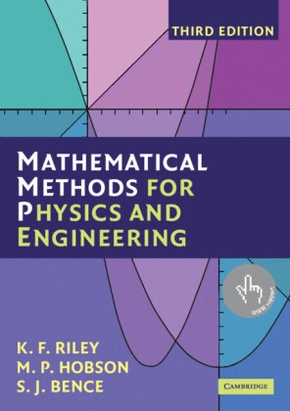# Mathematical Methods for Physics and Engineeringzum Preis von:
55,60€
Preis inkl. Mwst. versandkostenfrei

## A Comprehensive Guide

Kartoniert/Broschiert
Cambridge University Press, 2006, 1359 Seiten, Format: 17,4x24,7x5,6 cm, Artikeltyp: Englisches Buch, ISBN-10: 0521679710, EAN: 9780521679718, Bestell-Nr: 52167971EA

Sie möchten informiert werden, wenn dieser Artikel wieder lieferbar ist? Dann nutzen Sie unseren Info-Service per E-Mail. Sobald dieser oder ein Artikel in der selben Kategorie wieder lieferbar ist, erhalten Sie eine Nachricht.

### Reduzierte Artikel in dieser Kategorie

Produktbeschreibung

The third edition of this highly acclaimed undergraduate textbook is suitable for teaching all the mathematics for an undergraduate course in any of the physical sciences. As well as lucid descriptions of all the topics and many worked examples, it contains over 800 exercises. New stand-alone chapters give a systematic account of the'special functions'of physical science, cover an extended range of practical applications of complex variables, and give an introduction to quantum operators. Further tabulations, of relevance in statistics and numerical integration, have been added. In this edition, half of the exercises are provided with hints and answers and, in a separate manual available to both students and their teachers, complete worked solutions. The remaining exercises have no hints, answers or worked solutions and can be used for unaided homework.

#### Kurzbeschreibung:

This highly acclaimed undergraduate textbook teaches all the mathematics for undergraduate courses in the physical sciences.

#### Klappentext:

The third edition of this highly acclaimed undergraduate textbook is suitable for teaching all the mathematics for an undergraduate course in any of the physical sciences. As well as lucid descriptions of all the topics and many worked examples, it contains over 800 exercises. New stand-alone chapters give a systematic account of the 'special functions' of physical science, cover an extended range of practical applications of complex variables, and give an introduction to quantum operators. Further tabulations, of relevance in statistics and numerical integration, have been added. In this edition, half of the exercises are provided with hints and answers and, in a separate manual available to both students and their teachers, complete worked solutions. The remaining exercises have no hints, answers or worked solutions and can be used for unaided homework; full solutions are available to instructors on a password-protected web site, www.cambridge.org/9780521679718.

#### Inhaltsverzeichnis:

Prefaces; 1. Preliminary algebra; 2. Preliminary calculus; 3. Complex numbers and hyperbolic functions; 4. Series and limits; 5. Partial differentiation; 6. Multiple integrals; 7. Vector algebra; 8. Matrices and vector spaces; 9. Normal modes; 10. Vector calculus; 11. Line, surface and volume integrals; 12. Fourier series; 13. Integral transforms; 14. First-order ordinary differential equations; 15. Higher-order ordinary differential equations; 16. Series solutions of ordinary differential equations; 17. Eigenfunction methods for differential equations; 18. Special functions; 19. Quantum operators; 20. Partial differential equations: general and particular; 21. Partial differential equations: separation of variables; 22. Calculus of variations; 23. Integral equations; 24. Complex variables; 25. Application of complex variables; 26. Tensors; 27. Numerical methods; 28. Group theory; 29. Representation theory; 30. Probability; 31. Statistics; Index.

#### Rezension:

From reviews of previous editions: '...a great scientific textbook. It is a tour de force ... to write mathematical sections that are both complete and at an appropriate academic level. The authors have clearly succeeded in this challenge, making this a remarkable pedagogical book ... The choice of exercises is excellent and possibly the best feature of the book. In summary, this textbook is a great reference at undergraduate levels, particularly for those who like to teach or learn using lots of examples and exercises.' R. Botet, European Journal of Physics

### Alle Artikel in der Kategorie

ThemenArtikel

zum Preis von
(Preis inkl. Mwst.)